Refer to our Texas Go Math Grade 4 Answer Key Pdf to score good marks in the exams. Test yourself by practicing the problems from Texas Go Math Grade 4 Module 14 Assessment Answer Key.

Vocabulary

clockwise
counterclockwise
degree (°)
protractor

Choose the best term from the box.

Question 1.
The unit used to measure an angle is called a _________.
The unit used to measure an angle is called a degree.

Question 2.
________________ is the opposite of the direction in which the hands of a clock move.
Counterclockwise is the opposite of the direction in which the hands of a clock move.

Question 3.
A ______________ is a tool for measuring the size of an angle.
A protractor is a tool for measuring the size of an angle.

Concepts and Skills

Look at the shaded part of the circle. Tell what fraction of the circle the angle cuts out.

Question 4.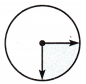$$\frac{1}{2}$$.

Explanation:
The fraction of the circle the angle cuts out is $$\frac{1}{2}$$.

Question 5.$$\frac{1}{4}$$.

Explanation:
The fraction of the circle the angle cuts out is $$\frac{1}{4}$$.

Question 6.$$\frac{1}{2}$$.

Explanation:
The fraction of the circle the angle cuts out is $$\frac{1}{2}$$.

Tell the measure of the angle in degrees.

Question 7.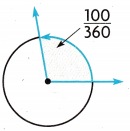10 degrees.

Explanation:
The measure of the angle in degrees is 10 degrees.

Question 8.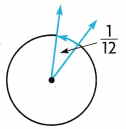30 degrees.

Explanation:
The measure of the angle in degrees is $$\frac{1}{12}$$×$$\frac{30}{30}$$ which is 30 degrees.

Use a protractor to draw the angle.

Question 9.
75°
The 75 degrees angle with the protractor is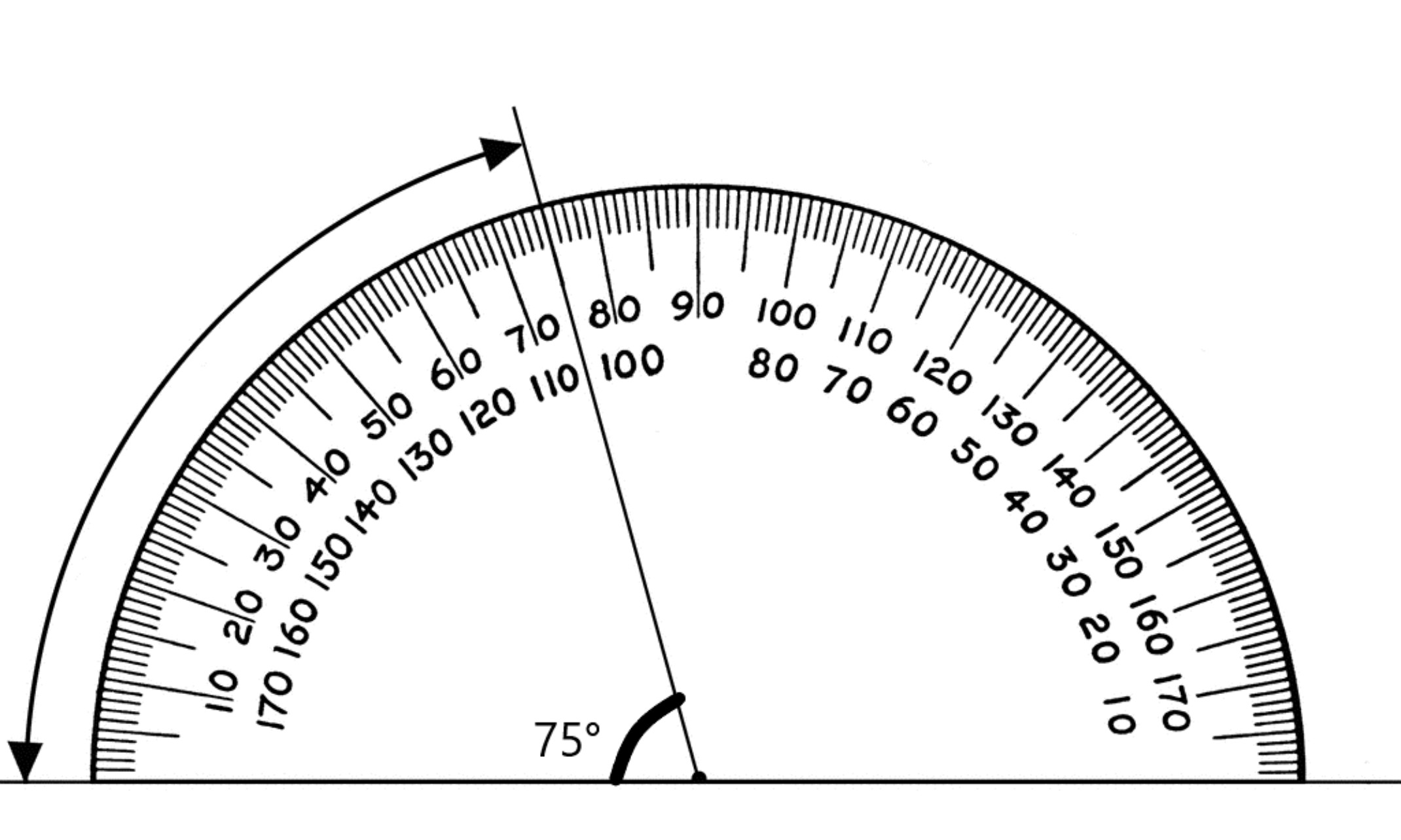Question 10.
127°
The 127° degrees angle with the protractor isQuestion 11.
What is the measure of the unknown angle in the figure?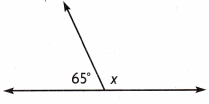(A) 25°
(B) 115°
(C) 125°
(D) 180°
B.

Explanation:
The measure of the unknown angle in the figure is X = 180 – 65 which is 115 degrees.

Which equation can you use to find the m∠ WRT?(A) 84° + 69° = __________
(B) 84°- 69° = __________
(C) 84° × 69° = __________
(D) 180° – 153° = __________
84° + 69° = 153°.

Explanation:
The equation is
m<WRT = 84° + 69° which is 153°.

Question 13.
Which best describes the m∠ CBT? Use a protractor to help you.(A) acute; 30°
(B) acute; 60°
(C) obtuse; 118°
(D) obtuse; 80°
D.

Explanation:
The angle m∠ CBT is obtuse 80°.

Question 14.
How many degrees are in an angle that cuts $$\frac{1}{360}$$ out of a circle?
(A) 0°
(B) 90°
(C) 1°
(D) 45°
The angle that cuts $$\frac{1}{360}$$ out of a circle is 1°